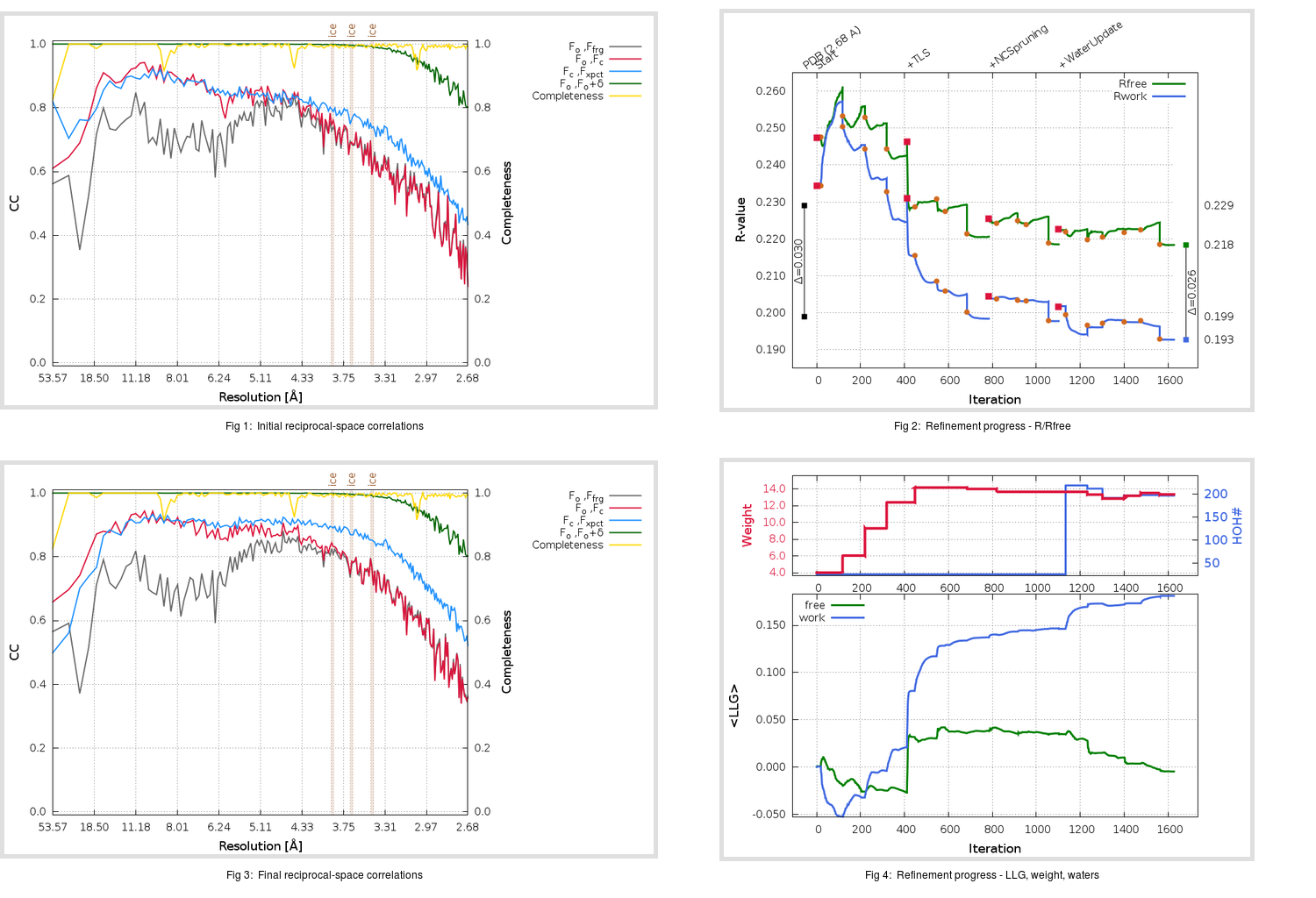Content:

```    Diffraction limits & principal axes of ellipsoid fitted to diffraction cut-off surface:
2.514         0.7149   0.0000  -0.6992       0.571 a* - 0.821 c*
2.951         0.0000   1.0000   0.0000       b*
2.935         0.6992   0.0000   0.7149       0.596 a* + 0.803 c*
```

## Deposited

` `
 Date deposited Date data collection Resolution R, Rfree 20200218 20200211 2.68 0.1970 0.2290

Molprobity (CCP4 7.0 version) summary:

```Ramachandran outliers =   0.00 %
favored =  95.53 %
Rotamer outliers      =   3.97 %
C-beta deviations     =     0
Clashscore            =   5.54
RMS(bonds)            =   0.0041
RMS(angles)           =   0.99
MolProbity score      =   2.07
Resolution            =   2.68
R-work                =   0.1970
R-free                =   0.2290
```

```Number of waters      =    26

<B> (all atoms) =  108.85 ( sd =   28.04 ) for      13180 non-hydrogen atoms
<B>   (protein) =  108.05 ( sd =   27.77 ) for      12782 non-hydrogen atoms
<B>     (water) =   82.32 ( sd =   14.01 ) for         26 non-hydrogen atoms
<B>    (others) =  138.01 ( sd =   20.66 ) for        372 non-hydrogen atoms

B min/max       (all non-hydrogen atoms) =   52.15 /  250.89
B min/max   (protein non-hydrogen atoms) =   52.15 /  250.89
B min/max     (water non-hydrogen atoms) =   60.44 /  116.90
B min/max     (other non-hydrogen atoms) =   75.04 /  196.78
```

## BUSTER (re-)refinement

` `

Molprobity (CCP4 7.0 version) summary:

```Ramachandran outliers =   0.13 %
favored =  96.43 %
Rotamer outliers      =   7.36 %
C-beta deviations     =     9
Clashscore            =   3.94
RMS(bonds)            =   0.0127
RMS(angles)           =   1.58
MolProbity score      =   2.08
Resolution            =   2.68
R-work                =   0.1927
R-free                =   0.2184
```

```Number of waters      =   196

<B> (all atoms) =  110.00 ( sd =   25.47 ) for      13350 non-hydrogen atoms
<B>   (protein) =  109.14 ( sd =   24.57 ) for      12782 non-hydrogen atoms
<B>     (water) =   91.83 ( sd =   15.68 ) for        196 non-hydrogen atoms
<B>    (others) =  149.27 ( sd =   25.85 ) for        372 non-hydrogen atoms

B min/max       (all non-hydrogen atoms) =   63.36 /  215.18
B min/max   (protein non-hydrogen atoms) =   65.99 /  211.66
B min/max     (water non-hydrogen atoms) =   63.36 /  215.18
B min/max     (other non-hydrogen atoms) =   81.10 /  190.98
```

Refinement progression:Results:

` `
 File Remark 6VW1_aB_refine.01_04_refine.pdb.gz exact refinement commands are in header 6VW1_aB_refine.01_04_refine.mtz.gz including original deposited data and several re-refinement map coefficients 6VW1_aB_refine.01_04_BUSTER_model.cif.gz including any non-standard compound restraints 6VW1_aB_refine.01_04_BUSTER_refln.cif.gz